# dtft properties

The discrete-time Fourier transform (DTFT) of a real, discrete-time signal x[n]  is a complex-valued function defined by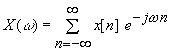where w is a real variable (frequency) and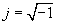. We assume x[n] is such that the sum converges for all w.

An important mathematical property is that X(w) is 2p-periodic in w,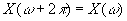, since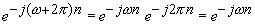for any (integer) value of n.

A plot of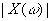vs w is called the magnitude spectrum of x[n], and a plot of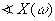vs w is called the phase spectrum of x[n]. These plots, particularly the magnitude spectrum, provide a picture of the frequency composition of the signal. Since X(w) is 2p-periodic, the magnitude and phase spectra need only be displayed for a 2p range in w, typically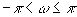.

The applet below illustrates properties of the discrete-time Fourier transform. You can sketch x[n] or select from the provided signals: a rectangular pulse and two one-sided exponential signals,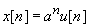, where u[n] is the unit step signal. (The exponentials continue for all n, that is, they are nonzero for all positive n. Sketched signals are assumed to be zero for all n outside the range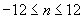.)

Sketch or select x[n] and click “show” in the top panel to display the corresponding spectra. Then choose an operation and click the corresponding “show” button to display the effects on the signal and its spectra.

Suppose y[n]  is the signal resulting from an operation on x[n], and let the discrete-time Fourier transform of y[n] be Y(w).The table below describes the operations available in the applet. For the ideal low pass filter, the cut-off frequency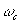specifies the highest frequency passed by the filter. For the ideal high pass filter,specifies the lowest frequency passed by the filter.

## Operation on x[n]

Resulting signal y[n]

Y(w),  -p < w £ p

Amplitude Scale

bx[n]

bX(w)

Time Shift

x[n – N]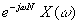Time Scale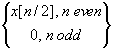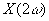Time Reverse

x[- n]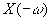First Difference

x[n] -  x[n-1]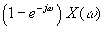Running Sum

x[n]+ x[n-1]+ x[n-2]+¼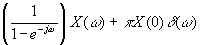Ideal Low Pass

filtered version of x[n],

cutoff frequency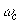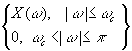Ideal High Pass

filtered version of x[n],

cutoff frequency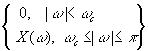return to demonstrations page

 Applet by Lan Ma.